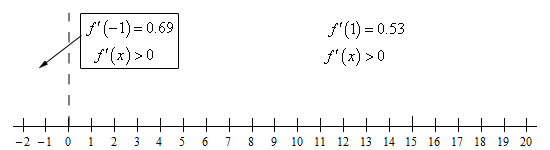Paul's Online Notes
Home / Calculus I / Derivatives / Chain Rule
Show Mobile Notice Show All Notes Hide All Notes
Mobile Notice
You appear to be on a device with a "narrow" screen width (i.e. you are probably on a mobile phone). Due to the nature of the mathematics on this site it is best views in landscape mode. If your device is not in landscape mode many of the equations will run off the side of your device (should be able to scroll to see them) and some of the menu items will be cut off due to the narrow screen width.

### Section 3.9 : Chain Rule

32. Determine where in the interval $$\left[ { - 1,20} \right]$$ the function $$f\left( x \right) = \ln \left( {{x^4} + 20{x^3} + 100} \right)$$ is increasing and decreasing.

Show All Steps Hide All Steps

Start Solution

We’ll first need the derivative because we know that the derivative will give us the rate of change of the function. Here is the derivative.

$f'\left( x \right) = \frac{{4{x^3} + 60{x^2}}}{{{x^4} + 20{x^3} + 100}} = \frac{{4{x^2}\left( {x + 15} \right)}}{{{x^4} + 20{x^3} + 100}}$

Note that we factored the numerator to help with the next step. In general, we don’t need to do this.

Show Step 2

Next, we need to know where the function is not changing and so all we need to do is set the derivative equal to zero and solve.

$\frac{{4{x^2}\left( {x + 15} \right)}}{{{x^4} + 20{x^3} + 100}} = 0\hspace{0.25in} \to \hspace{0.25in} 4{x^2}\left( {x + 15} \right) = 0\hspace{0.25in} \Rightarrow \hspace{0.25in} x = 0,\,\,\,\,x = - 15$

Note, that in general, we also need to be concerned with where the derivative is not defined as well. Functions can (and often do) change sign where they are not defined. In this case however we’ve restricted the interval down to a range where the function exists and is continuous on the given interval and so this is something we need to worry about for this problem.

In the next Chapter we will start also looking at what happens if the derivative is also not defined at particular points.

Note as well that we really should at this point step back and recall that we are working on the interval $$\left[ { - 1,20} \right]$$. We are only interested in what is happening on this interval and so we should make sure that the points found above are inside the interval.

In this case only $$x = 0$$is in the interval and so we’ll need to exclude $$x = - 15$$ from our work for the rest of this problem.

Show Step 3

To get the answer to this problem all we need to know is where the derivative is positive (and hence the function is increasing) or negative (and hence the function is decreasing). Because the derivative is continuous we know that the only place it can change sign is where the derivative is zero. So, as we did in this section a quick number line will give us the sign of the derivative for the various intervals.

Here is the number line for this problem.So, we can see that, in this case function is increasing everywhere in the interval $$\left[ { - 1,20} \right]$$ except $$x = 0$$. Recall that at this point the derivative was zero and hence the function is not changing (and therefore can’t be increasing).

So, the formal answer for this problem is,

$\require{bbox} \bbox[2pt,border:1px solid black]{{{\mbox{Increasing :}}\, - 1 \le x < 0,\,\,\,\,\,0 < x \le 20}}$

Note that because we’ve only looked at what is happening in the interval $$\left[ { - 1,20} \right]$$ we can’t say anything about the increasing/decreasing nature of the function outside of this interval.